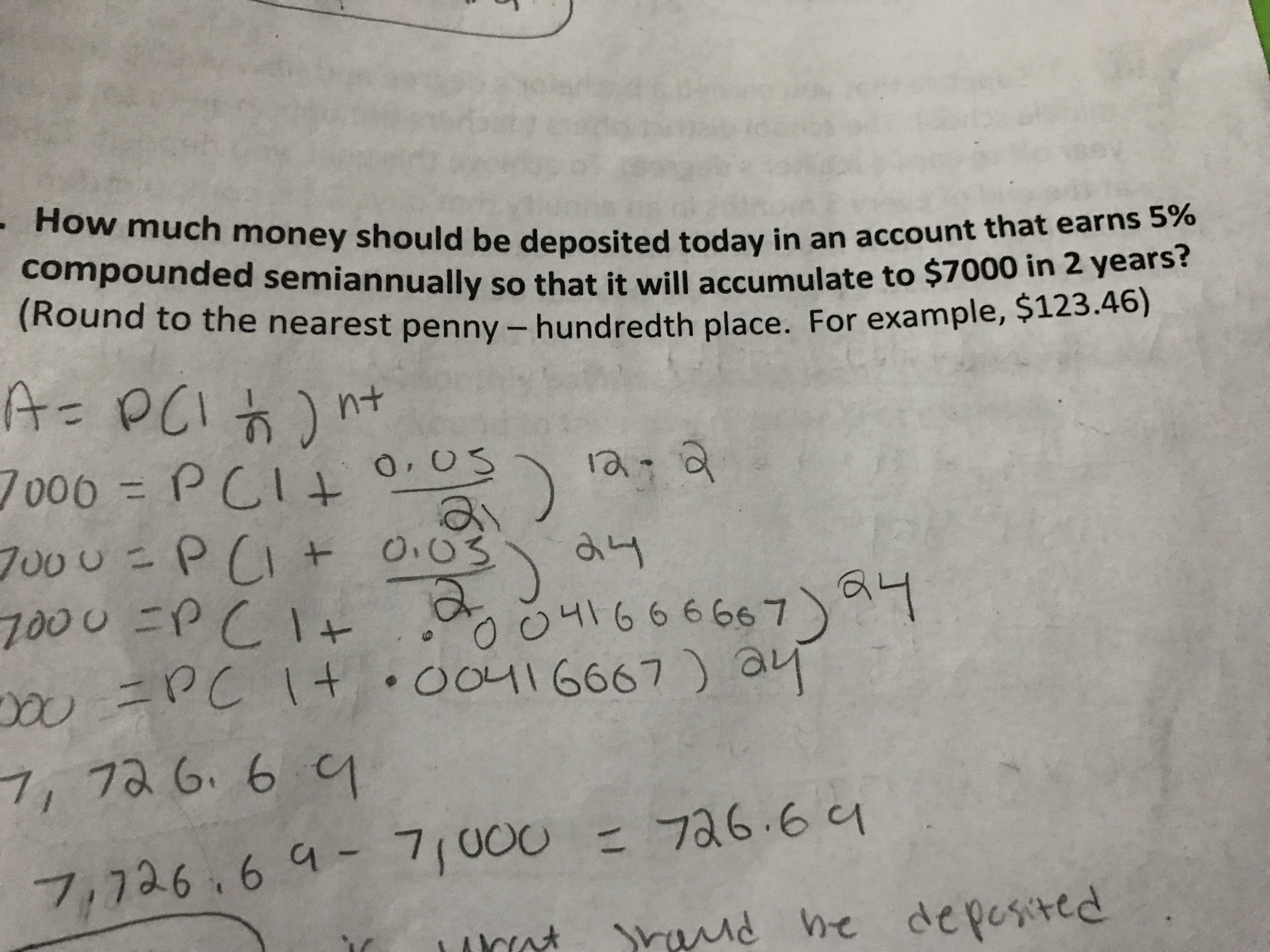# How much money should be deposited today in an account that earns 5%compounded semiannually so that it will accumulate to \$7000 in 2 years?(Round to the nearest pen ny - hundredth place. For example, \$123.46)A= PCI)7006 PCIntCI+a a700U P(I 0.037000 PCIPC it 00116667) ayOO41666667OOL7, 7a 6. 6 C7a6.67,726 64- 7/000ndhe depested

Question
1 viewshelp_outlineImage TranscriptioncloseHow much money should be deposited today in an account that earns 5% compounded semiannually so that it will accumulate to \$7000 in 2 years? (Round to the nearest pen ny - hundredth place. For example, \$123.46) A= PCI) 7006 PCI nt CI+ a a 700U P(I 0.03 7000 PCI PC it 00116667) ay OO41666667 OOL 7, 7a 6. 6 C 7a6.6 7,726 64- 7/000 nd he depested fullscreen
check_circle

Step 1

Let P be the amount to be deposited today.

r = rate per annum = 5%

m = frequency of compunding in a year = semi annual compounding = 2 times in a year

n = number of years = 2

A = Accumulated value = \$ 7,000

Step 2

Hence, the conecting equation is:

A = 7,000 = P x (1 + r / m)n x m = P x (1 + 5% / 2)2 x 2 = 1.1038P

Hen...

### Want to see the full answer?

See Solution

#### Want to see this answer and more?

Solutions are written by subject experts who are available 24/7. Questions are typically answered within 1 hour.*

See Solution
*Response times may vary by subject and question.
Tagged in